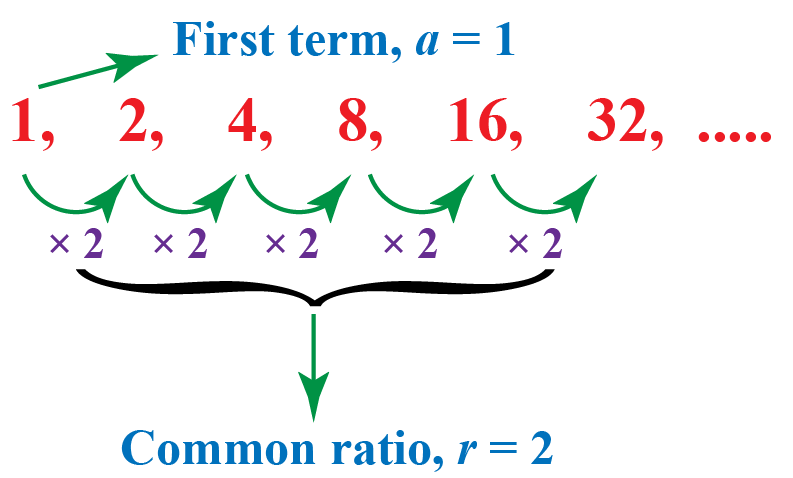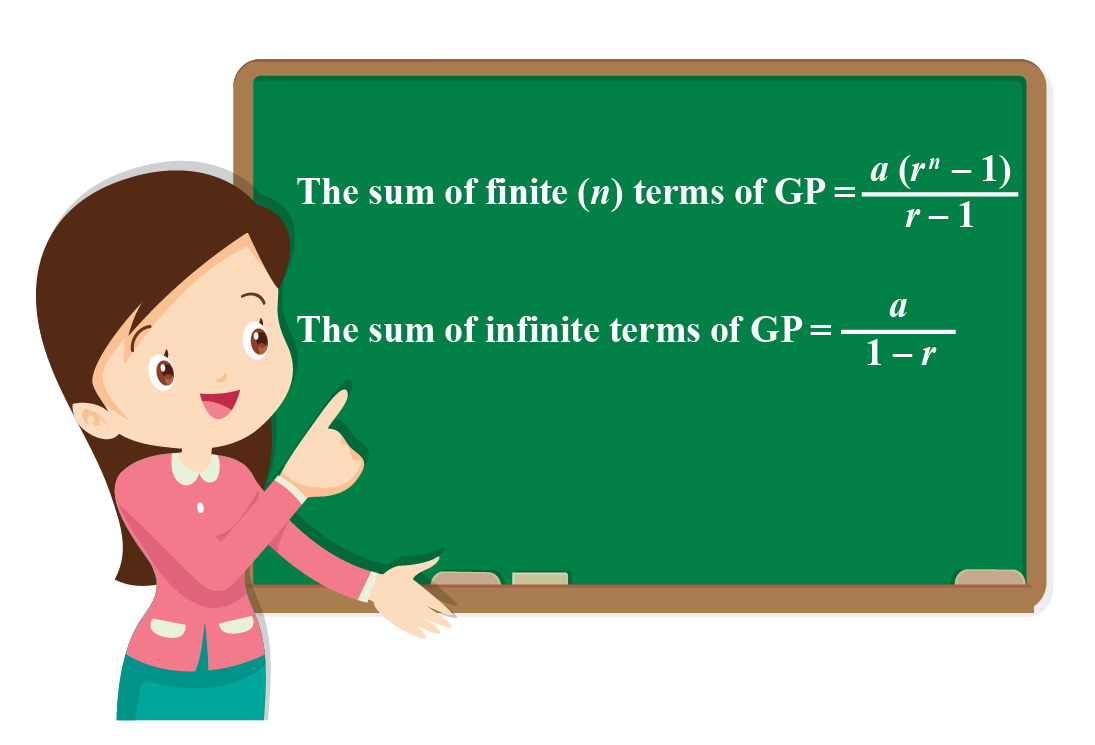# Sum of an Infinite GP

Sum of an Infinite GP

 1 What is a Geometric Progression (GP)? 2 Sum of n terms of Geometric Progression 3 Sum of Infinite Geometric Progression 4 Think Out of the Box! 5 Solved Examples on Sum of GP 6 Sum of GP Calculator 7 Practice Questions 8 Challenging Questions on Sum of GP 9 Maths Olympiad Sample Papers 10 Frequently Asked Questions (FAQs)

We at Cuemath believe that Math is a life skill. Our Math Experts focus on the “Why” behind the “What.” Students can explore from a huge range of interactive worksheets, visuals, simulations, practice tests, and more to understand a concept in depth.

Book a FREE trial class today! and experience Cuemath’s LIVE Online Class with your child.

## What is a Geometric Progression (GP)?

A sequence of numbers in which the ratio of successive terms is constant is said to be a geometric progression (GP) or a geometric sequence.

In a geometric sequence, every term is obtained by multiplying its previous term by a constant.

For example, $$1,2,4,8,16,32, \ldots$$ is a GP as every term is obtained by multiplying its previous term by a constant 2Here, the first term is $$a=1$$

The common ratio is $$r=2$$

## Sum of n terms of Geometric Progression

We will now discuss how to derive the formula for the sum of $$n$$ terms of an arbitrary GP.

Consider the sum of the first $$n$$ terms of a GP with first term $$a$$ and common ratio $$r$$:

$S=a+a r+a r^{2}+\ldots+a r^{n-1}$

Multiply both sides by $$r$$ and write the terms with the same power of $$r$$ below each other as shown below:

$\begin{array}{r} S=a+a r+a r^{2}+\ldots+a r^{n-1} \\ r S=a r+a r^{2}+\ldots+a r^{n-1}+a r^{n} \end{array}$

Now, subtract the first relation from the second relation:

$\begin{array}{l} (r-1) S=a r^{n}-a \\ \Rightarrow S=\dfrac{a\left(r^{n}-1\right)}{r-1} \end{array}$

Thus, the formula for the sum of $$n$$ terms of a GP is:

 $S=\frac{a\left(r^{n}-1\right)}{r-1}$

### Example:

A truck transports 500 kg of cement to the market.

The quantity of cement that the truck transports increases by 20% every day.

What is the total quantity of cement that the truck transports in a week to the market?

Round the answer to the nearest whole number.Solution:

The initial quantity of cement is $$a=500$$ kg

Since the amount increases by 20% every day, the common ratio is $$r=1.20$$

The number of days in a week is $$n=7$$

The total quantity of cement transported in one week is found using the formula for sum of $$n$$ terms of a GP.

\begin{align}S&=\dfrac{a\left(r^{n}-1\right)}{r-1}\\[0.3cm] &= \dfrac{500(1.20^7-1)}{1.20-1}\\[0.3cm] & \approx 6458 \text{ kg} \end{align}

Thus,

Total quantity of cement = 6458 kg

## Sum of Infinite Geometric Progression

Consider the following sum:

$S = 1 + \frac{1}{2} + \frac{1}{4} + \frac{1}{8} + ...\,\,\,{\rm{infinite\,\, terms}}$

Will the sum be finite or infinite?

This is a GP with the common ratio of magnitude less than 1

It turns out that all such GPs have finite sums.

Let us find out the sum in this particular case.

We follow the same approach as earlier.

We take the original series for S, and multiply it by the common ratio, and write the equal terms below each other.

Then, we subtract the two series:

\begin{align}S& = 1 + \frac{1}{2} + \frac{1}{4} + \frac{1}{8} + ...\,\,\,{\rm{infinite \,\, terms}}\\[0.2cm] \frac{S}{2}& = \frac{1}{2} + \frac{1}{4} + \frac{1}{8} + ...\,\,\,{\rm{infinite\,\, terms}}\,\,\\[0.2cm] &\Rightarrow \,\,\,S - \frac{S}{2} = 1\,\,\, \Rightarrow \,\,\,\frac{S}{2} = 1\,\,\, \Rightarrow \,\,\,S = 2\end{align}

When we subtract the two series, only the first term is left, since by virtue of being infinite series, the other terms all cancel out.

Let us generalize this.

Consider a GP with first term equal to a and common ratio r such that $$\left| r \right| < 1$$

The GP will have a finite sum (this can be proved rigorously, but we won’t go into that here).

Let us represent the sum (for infinitely many terms) of this series by $${S_\infty }$$

We have:

\begin{align}\;\;{S_\infty }& = a + ar + a{r^2} + a{r^3}...\,\,\,{\rm{to }}\,\,\infty \\[0.2cm]r{S_\infty } &= ar + a{r^2} + a{r^3}...\,\,\,{\rm{to }}\,\,\infty \\[0.2cm] &\Rightarrow \,\,\,{S_\infty } - r{S_\infty } = a\\[0.2cm] &\Rightarrow \,\,\,\left( {1 - r} \right){S_\infty } = a\,\,\, \Rightarrow \,\,\,{S_\infty } = \frac{a}{{1 - r}}\end{align}

Thus, the formula for the infinite terms of a GP is:

 $S = \frac{a}{1 - r}$

Here are the formulas for the sum of finite and infinite terms of a GP.Think Tank
1. The sum of infinite terms of a GP with first term $$\mathbf{a}$$ and the common ratio $$\mathbf{r}$$ is,
$S = \dfrac{a}{1 - r}$
Does this formula work even when $$\mathbf{r \geq 1}$$?

Help your child score higher with Cuemath’s proprietary FREE Diagnostic Test. Get access to detailed reports, customised learning plans and a FREE counselling session. Attempt the test now.

## Solved Examples

 Example 1

Find the following sums:

(a) $$S = \frac{1}{3} + \frac{1}{9} + \frac{1}{{27}} + ...\,\,{\rm{to }}\;\infty$$

(b) $$S = 1 - \frac{1}{2} + \frac{1}{4} - \frac{1}{8} + ...\,\,\,{\rm{to }}\;\infty$$

Solution:

(a) We have:

$a = \frac{1}{3},\,\,\,r = \frac{1}{3}$

Thus,

$S = \frac{a}{{1 - r}} = \frac{{\frac{1}{3}}}{{1 - \frac{1}{3}}} = \frac{1}{2}$

(b) In this case, we have:

$a = 1,\,\,\,r = - \frac{1}{2}$

Thus,

$S = \frac{a}{{1 - r}} = \frac{1}{{1 - \left( { - \frac{1}{2}} \right)}} = \frac{2}{3}$

 $$\therefore$$ \begin{align} (a)\,\, S &= \frac{1}{2}\0.2cm] (b)\,\, S &=\frac{2}{3} \end{align}  Example 2 Jibin deposited Rs 1,00,000 in a bank in the month of January where the money increases by 15% every month.What is the total amount of money in his account at the end of December? Round your answer to the nearest whole number. Solution: The initial amount of money deposited is $$a=1,00,000$$ Since the amount increases by 15% every day, the common ratio is $$r=1.15$$ The number of months from January to December is $$n=12$$ The total amount in Jibin's account can be found using the formula for sum of $$n$$ terms of a GP. \[\begin{align}S&=\frac{a\left(r^{n}-1\right)}{r-1}\\[0.3cm] &= \dfrac{100000(1.15^{12}-1)}{1.15-1}\\[0.3cm] & \approx 2900167 \end{align}

 $$\therefore$$ Total amount = Rs. 29,00,167
 Example 3

Find the following sum:

$S = \frac{1}{3} + \frac{1}{{{5^2}}} + \frac{1}{{{3^3}}} + \frac{1}{{{5^4}}} + \frac{1}{{{3^5}}} + \frac{1}{{{5^6}}} + ...\,\,\,{\rm{to }}\,\,\infty$

Solution:

We can divide the given series into two separate GPs:

\begin{align}&{S_1} = \frac{1}{3} + \frac{1}{{{3^3}}} + \frac{1}{{{3^5}}} + ... = \frac{{\frac{1}{3}}}{{1 - \frac{1}{{{3^2}}}}} = \frac{{\frac{1}{3}}}{{\frac{8}{9}}} = \frac{3}{8}\\&{S_2} = \frac{1}{{{5^2}}} + \frac{1}{{{5^4}}} + \frac{1}{{{5^6}}} + ... = \frac{{\frac{1}{{{5^2}}}}}{{1 - \frac{1}{{{5^2}}}}} = \frac{{\frac{1}{{25}}}}{{\frac{{24}}{{25}}}} = \frac{1}{{24}}\end{align}

Thus,

$S = {S_1} + {S_2} = \frac{3}{8} + \frac{1}{{24}} = \frac{5}{{12}}$

## 2. What is the sum of n terms in GP?

The sum of $$n$$ terms of a geometric series whose first term is $$a$$ and the common ratio is $$r$$ is found by using the formula:

$S=\frac{a\left(r^{n}-1\right)}{r-1}$

## 3. How do you find the sum of infinite values?

It may not be possible to find the sum of infinite values all the time.

But we can find the sum of an infinite geometric series whose first term is $$a$$ and the common ratio is $$r$$ by using the formula:

$S = \frac{a}{1 - r}$

More Important Topics
Numbers
Algebra
Geometry
Measurement
Money
Data
Trigonometry
Calculus
More Important Topics
Numbers
Algebra
Geometry
Measurement
Money
Data
Trigonometry
Calculus